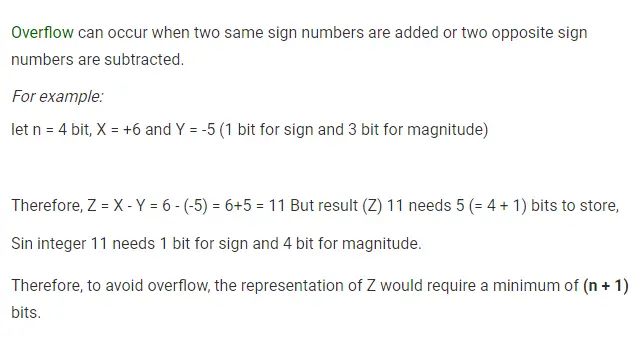# Gate CS-2019 Question Paper With Solutions

Q. 15 Consider Z = X − Y where X, Y and Z are all in sign-magnitude form. X and Y are each represented in n bits. To avoid overflow, the representation of Z would require a minimum of:

(A) n bits

(B) n−1 bits

(C) n+1 bits

(D) n+2 bits